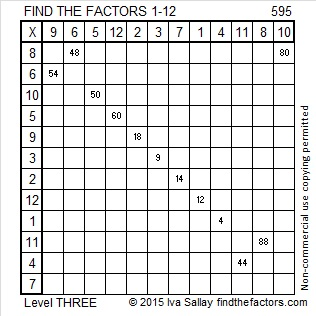# 595 and Level 3

595 = (34/2)(34 + 1) so 595 is the 34th triangular number.

Thus 1 + 2 + 3 + 4 + . . . . . + 31 + 32 + 33 + 34 = 595

595 is also the hypotenuse of four Pythagorean triples. Each of these triples has its own greatest common factor.

• 91-588-595
• 252-539-595
• 280-525-595
• 357-476-595Print the puzzles or type the solution on this excel file: 12 Factors 2015-08-24

—————————————————————————————————

• 595 is a composite number.
• Prime factorization: 595 = 5 x 7 x 17
• The exponents in the prime factorization are 1, 1, and 1. Adding one to each and multiplying we get (1 + 1)(1 + 1)(1 + 1) = 2 x 2 x 2 = 8. Therefore 595 has exactly 8 factors.
• Factors of 595: 1, 5, 7, 17, 35, 85, 119, 595
• Factor pairs: 595 = 1 x 595, 5 x 119, 7 x 85, or 17 x 35
• 595 has no square factors that allow its square root to be simplified. √595 ≈ 24.39262.—————————————————————————————————

A Logical Approach to solve a FIND THE FACTORS puzzle: Find the column or row with two clues and find their common factor. Write the corresponding factors in the factor column (1st column) and factor row (top row).  Because this is a level three puzzle, you have now written a factor at the top of the factor column. Continue to work from the top of the factor column to the bottom, finding factors and filling in the factor column and the factor row one cell at a time as you go.